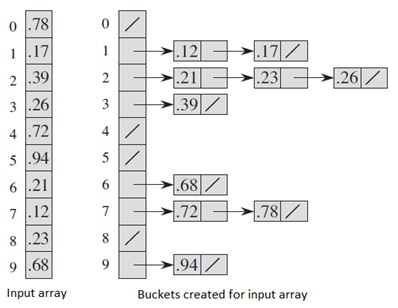# Program for Bucket Sort in Kotlin

In this article, we are going to learn how to implement bucket sort in Kotlin? Here, you will find what is bucket sort, its algorithm and program in kotlin.
Submitted by Aman Gautam, on December 20, 2017

Bucket sort algorithm sorts the elements which are uniformly distributed. Like in counting sort, we assume that the input elementsarein small range, here we assume that elements are uniformly distributed over interval [0,1] so they both are fast. Both have little idea about the input they are going to process.

Bucket sort distributes the elements in the buckets and then separately sort each bucket using insertion sort. Since the elements are uniformly distributed so not many elements would fall in each bucket. So we would simply sort each bucket and then combine them to produce the result.Image source: Introduction to Algorithms, book by Thomas H. Cormen, Charles E. Leiserson, Ronald L. Rivest, and Clifford Stein(CLRC)

Assume If the elements are not uniformly distributed then all elements can fall in a single bucket and then If we sort them using insertion sort then this can take up to O(n2) time as usual by insertion sort. There would be no meaning of using bucket sort.

Here are few assumptions before using Bucket sort,

1. Elements are in range [0,1) // not including 1.
2. Elements are uniformly distributed over the range.

Algorithm

```	    BUCKET_SORT(A)
1.	let B[0..n-1] be a new array
2.	for I = 0 to n-1
3.	make B[i] an empty list
4.	for i=1 to n
5.	insert a[i] into b[floor(n*a[i])]
6.	for I = 0 to n-1
7.	sort b[i] using insertion sort
8.	concatenate list b,b…b[n-1] together in order

//Algorithm source from Introduction to Algorithms by CLRC
```

Complexity O(n+k)

```fun insertion_sort(A: ArrayList<Double>) {
var n = A.size
var i: Int
for (j in 1 until n) {
var key = A[j]
i = j - 1
while (i >= 0 && A[i] > key) {
A[i + 1] = A[i]
i--
}
A[i + 1] = key
}
}

fun bucket_sort(A:Array<Double>){
var n=A.size
var bucket = Array<ArrayList<Double>>(n,{i-> ArrayList() })   // creating buckets with n size
for(i in 0..A.size-1){
}
for(i in 0..A.size-1){
insertion_sort(bucket[i])                               // sorting a list using inserton sort
}
for (i in 1..A.size-1){
bucket.addAll(bucket[i])                              // concatenate all the buckets
}
println("After sorting :")
for (i in bucket)
{
print("\$i ")                                            // print the sorted elements
}
}
fun main(arg: Array<String>) {
print("Enter no. of elements :")

println("Enter elements : ")
var A = Array(n, { 0.0 })
for (i in 0 until n)

bucket_sort(A)

}
```

Output

```Enter no. of elements :10
Enter elements :
0.32
0.43
0.53
0.56
0.87
0.98
0.76
0.68
0.57
0.28
After sorting :
0.28 0.32 0.43 0.53 0.56 0.57 0.68 0.76 0.87 0.98
```

What's New

Top Interview Coding Problems/Challenges!

Languages: » C » C++ » C++ STL » Java » Data Structure » C#.Net » Android » Kotlin » SQL
Web Technologies: » PHP » Python » JavaScript » CSS » Ajax » Node.js » Web programming/HTML
Solved programs: » C » C++ » DS » Java » C#
Aptitude que. & ans.: » C » C++ » Java » DBMS
Interview que. & ans.: » C » Embedded C » Java » SEO » HR
CS Subjects: » CS Basics » O.S. » Networks » DBMS » Embedded Systems » Cloud Computing
» Machine learning » CS Organizations » Linux » DOS
More: » Articles » Puzzles » News/Updates